Next: Electric Field of a Up: Gauss' Law Previous: Electric Field-Lines

Gauss' Law

One of the most useful results in electrostatics is named after the celebrated German mathematician Karl Friedrich Gauss (1777-1855).

Suppose that a positive point charge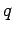generates an electric field. Consider a spherical surface of radius, centred on the charge. The normal to this surface is everywhere parallel to the direction of the electric field, since the field always points radially away from the charge. The area of the surface is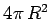. Finally, the strength of the electric field at radiusis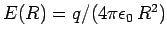. Hence, if we multiply the electric field-strength by the area of the surface, we obtain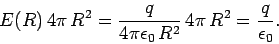(66)

Note that the final result is independent of the radius of the sphere. Thus, the same result would be obtained for any sphere centred on the charge. This is the essence of Gauss' law.

You may be wondering why it took a famous German mathematician to prove such a trivial-seeming law. Well, Gauss proved that this law also applies to any closed surface, and any distribution of electric charges. Thus, if we multiply each outward element of a general closed surfaceby the component of the electric field normal to that element, and then sum over the entire surface, the result is the total charge enclosed by the surface, divided by. In other words,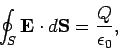(67)

whereis a closed surface, and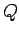is the charge enclosed by it. The integral is termed the electric flux,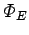, through the surface, and is proportional to the number of electric field-lines which pierce this surface. We adopt the convention that the flux is positive if the electric field points outward through the surface, and negative if the field points inward. Thus, Gauss' law can be written:
The electric flux through any closed surface is equal to the total charge enclosed by the surface, divided by.

Gauss' law is especially useful for evaluating the electric fields produced by charge distributions which possess some sort of symmetry. Let us examine three examples of such distributions.Next: Electric Field of a Up: Gauss' Law Previous: Electric Field-Lines
Richard Fitzpatrick 2007-07-14